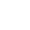/    2018-08-07

Spark RDD介绍

RDD是Resilient Distributed Dataset，中文翻译是弹性分布式数据集。该类是Spark是核心类成员之一，是贯穿Spark编程的始终。

Spark-shell下实现对本地文件的单词统计

1. 思路

word count是大数据学习的经典案例，很多功能实现都可以归结为是word count的使用。

2.处理流程3.分步实现代码

```// 进入spark shell环境
\$>spark-shell

// 1.加载文件
scala>val rdd1 = sc.textFile("/homec/centos/1.txt")

// 2.压扁每行
scala>val rdd2 = rdd1.flatMap(_.split(" "))

// 3.标1成对
scala>val rdd3 = rdd2.map(w=>(w,1))

// 4.按照key聚合每个key下的所有值
scala>val rdd4 = rdd3.reduceByKey(_+_)

// 5.显式数据
scala>rdd4.collect()```

4.一步实现代码

```\$scala>sc.textFile("file:///home/centos/1.txt")
.flatMap(_.split(" "))
.map((_,1))
.reduceByKey(_+_)
.collect```

Spark-shell下实现对气温数据的最大最小聚合

1.思路分析

2.处理流程3.分步实现代码

```// 进入spark shell环境
\$>spark-shell

// 1. 加载气温数据文件
scala>val rdd1 = sc.textFile("/home/centos/temps.dat")

// 2.压扁每行
scala>val rdd2 = rdd1.flatMap(e=>{
val arr = e.split(" ")
(arr(0).toInt, (arr(1).toInt ,arr(1).toInt))
})

// 3.reduceByKey
scala>val rdd3 = rdd2.reduceByKey((a,b)=>{
import scala.math
(math.max(a(0),b(0)) ,math.min(a(1),b(1))
})

// 4.收集日志
scala>rdd3.collect()```

4.一步实现代码

```\$scala>sc.textFile("file:///home/centos/temps.dat")
.map(line=>{
val arr = line.split("")
(arr(0).toInt,(arr(1).toInt , arr(1).toInt))
})
.reduceByKey((a,b)=>{
import scala.math
(math.max(a(0) , b(0)) , math.min(a(1) , b(1)))
})
.collect()```(6)